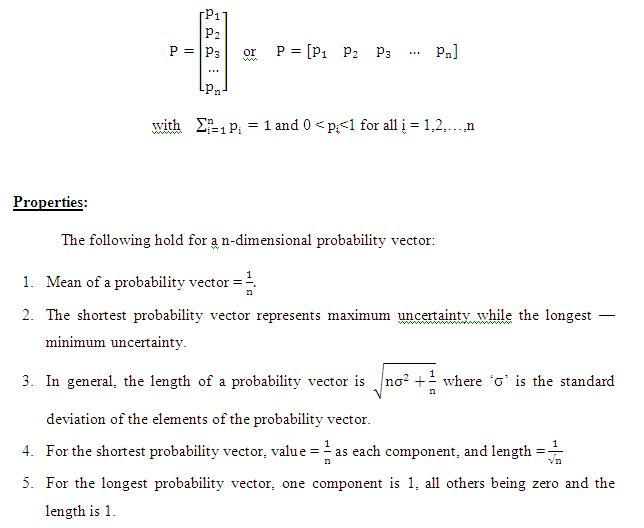# Probability Vector

Posted in Statistics, Total Reads: 6839

## Definition: Probability Vector

A probability vector is a vector (a column or row matrix) which is non-negative and all elements adding upto unity. The elements of a probability vector give us the outcomes which can occur, of a discrete random variable and the vector as a whole represents the probability mass function of that random variable. Probability vector is a convenient, compact notation for denoting the behaviour of a discrete random variable.

Mathematically, if p1, p2, p3, …, pn be a set of ‘n’ probabilities of a variable, then its probability vector will be:Browse the definition and meaning of more terms similar to Probability Vector. The Management Dictionary covers over 7000 business concepts from 6 categories. This definition and concept has been researched & authored by our Business Concepts Team members.

Search & Explore : Management Dictionary

Similar Definitions from same Category: